IntelliJ IDEA Tutorials Videos

# Tutorial: Spock Part 3 – Data Driven Testing

In Part 3 of our Spock tutorial, we’ll look at Data Driven Testing. This is one of my favourite things about Spock, although it is also supported in other frameworks like JUnit 5.

Tutorial: Spock

These blog posts cover the same material as the video. This provides an easy way for people to skim the content quickly if they prefer reading to watching, and to give the reader/watcher code samples and links to additional information.

## Data Pipes

When we’re testing a particular path, we sometimes want to check that a known set of values leads to the same result.

The exception test we just wrote is a good example – we know there’s more than one input which should cause this exception to be thrown, and we might want to test all of them. In our case, any integer that is less than three should cause the exception. When you’re using tests to document the expected behaviour, it’s helpful to add the full list of values that can cause the Exception, or at least a sample list that demonstrates our expectations. Create a new test method that uses Data Pipes to do this:

``````def "should expect an Exception to be thrown for a number of invalid inputs"() {
when:
new Polygon(sides)

then:
def exception = thrown(TooFewSidesException)
exception.numberOfSides == sides

where:
sides << [-1, 0, 1, 2]
}``````

Note the new label at the end, `where`, which specifies the input values to the test. This test runs multiple times with different values passed into the constructor. So instead of passing in zero, it passes in a variable `sides`. The assertion also needs to check the `numberOfSides` on the exception matches the same number that we passed into the constructor.

The variable `sides` is defined in the where `block`. This uses the left-shift operator (`<<`) to give a list of values that we want `sides` to be.

``````where:
sides << [-1, 0, 1, 2]``````

There are a couple of Groovy things to note here:

The `where` block says “run this test with each of the following values: a negative value, zero, one and two”.

Run this test to see what happens.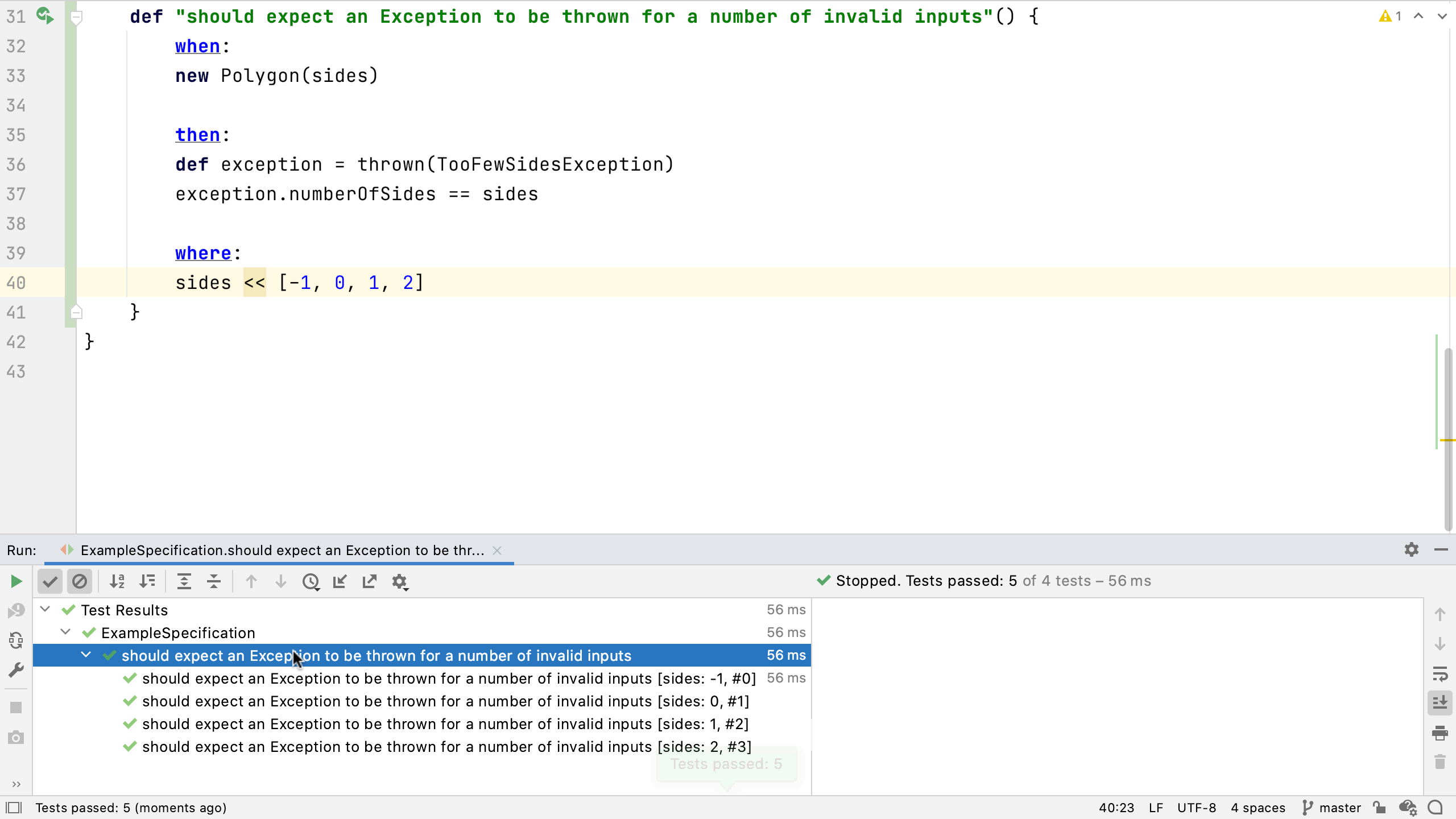The test is effectively run four different times, the whole test is run once per value in that list for `sides`. IntelliJ IDEA shows the name of the test, then underneath that the test name plus the value of `sides` for each of the four values. All four of these runs passed, because our code correctly throws the expected Exception for each of these values.

If we want, we can change the method name to make it easier to understand what’s being tested. We can use hash and the name of a data variable in the method name to create a true description.

``def "should expect an Exception to be thrown for invalid input: #sides"() {``

Re-run this, and IntelliJ IDEA will show this updated method name with the value of “sides”, and no extra noise.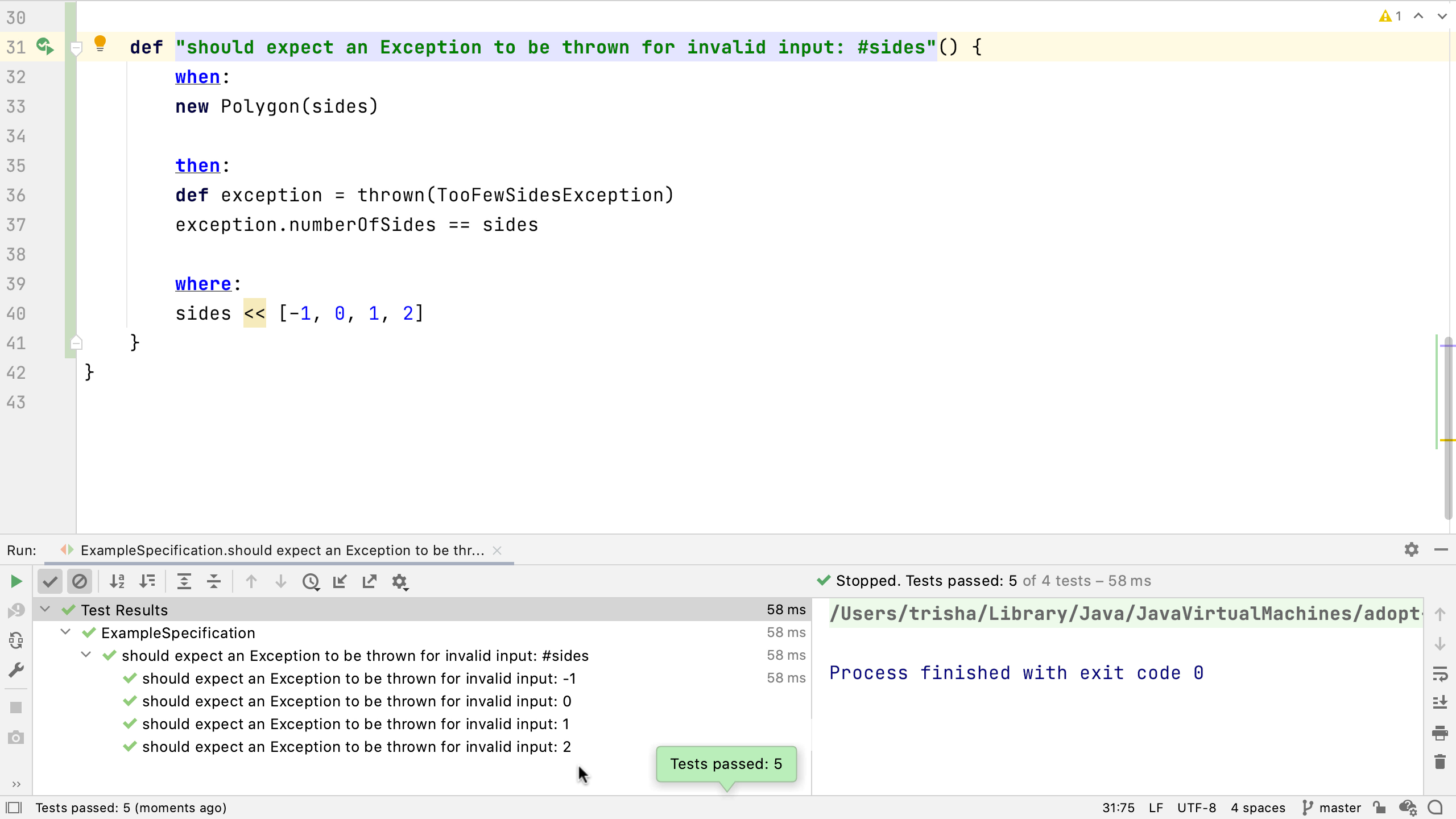(Note: this is the behaviour in the latest versions of Spock. If you don’t see this behaviour, you may need to use the @Unroll annotation on your method).

Let’s look at what happens if one of these values causes the test to fail. We know this exception should be thrown for a number of sides that’s two or fewer so let’s change one value to three.

``````def "should expect an Exception to be thrown for invalid input: #sides"() {
when:
new Polygon(sides)

then:
def exception = thrown(TooFewSidesException)
exception.numberOfSides == sides

where:
sides << [-1, 0, 3, 2]
}``````

Run the test to see one of the great things about data driven testing – all the tests are run even if one of the tests fails.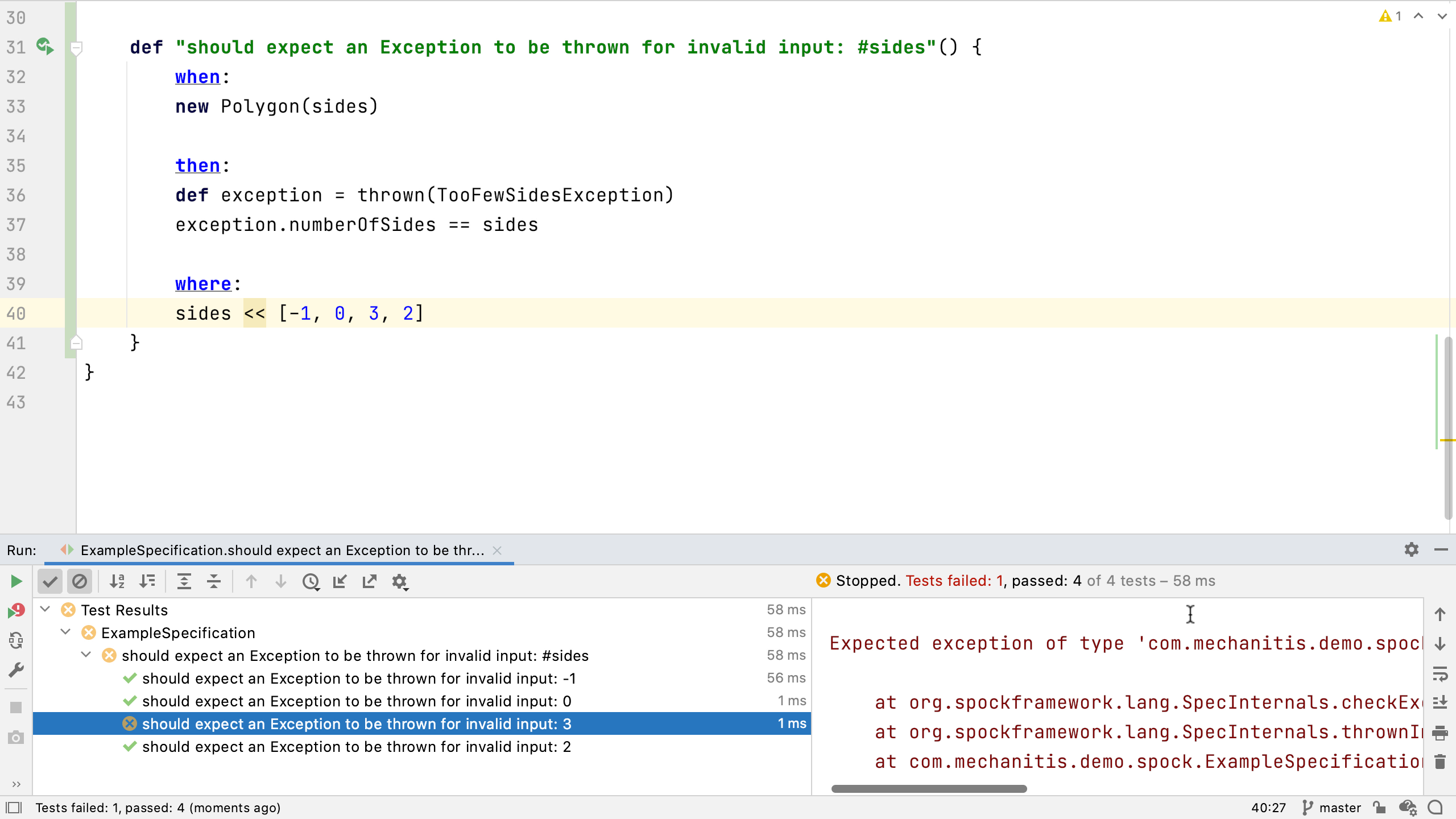So we can see clearly which cases pass and which fail. If one of them fails, we can see what caused the problem. In our case, the test was expecting an Exception to be thrown and it wasn’t. Go back and fix the test by replacing the `3` with a `1`.

Data pipes aren’t just for testing exceptional cases. We might want to use them to test a series of valid inputs.

Create another test:

``````def "should be able to create a polygon with #sides sides"() {
when:
def polygon = new Polygon(sides)

then:
polygon.numberOfSides == sides

where:
sides << [3, 4, 5, 8, 14]
}``````

Once again the test creates a polygon with a specified number of sides. Then it checks that the number of sides is the expected value. The `sides` variable is set up with a whole list of valid values. Running this test shows something similar to the previous test – a passing test for each of the values for `sides`.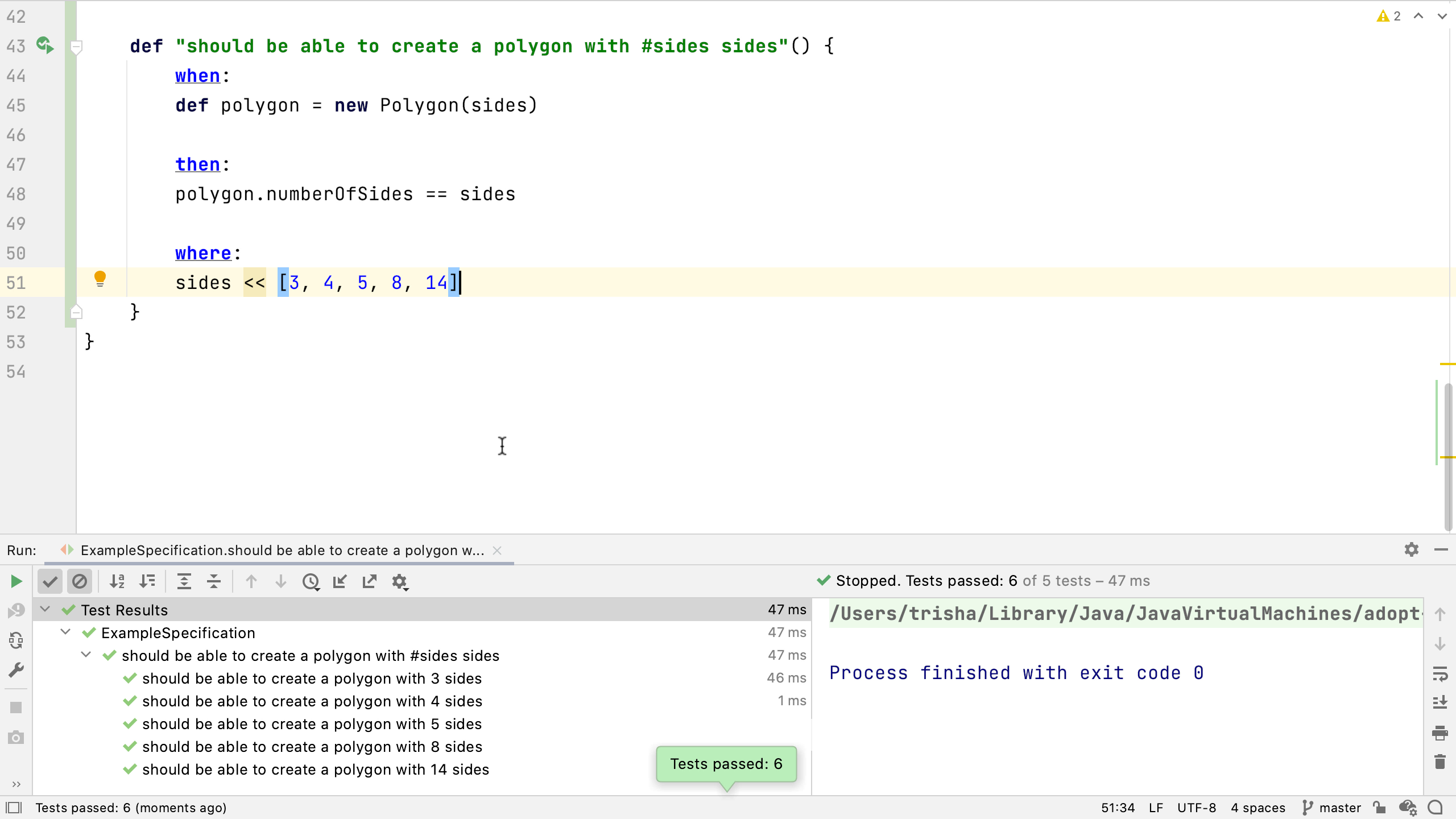This test is quite a simple one, and we can reduce the amount of code and do the same thing. We can inline the creation of the Polygon (by pressing ⌘⌥N (macOS), or Ctrl+Alt+N (Windows/Linux) on the `polygon` variable name), so the constructor is called in the same line as the assertion. If we just have one statement which is setup, test, and assertion, we can use the `expect` label like we did in our very first simple assertion test. Of course, we still need the `where` block as this sets all the expected values for number of sides.

``````def "should be able to create a polygon with #sides sides"() {
expect:
new Polygon(sides).numberOfSides == sides

where:
sides << [3, 4, 5, 8, 14]
}``````

View steps in video

## Data Tables

Data pipes are a nice way to specify a limited set of data to test. Spock also supports Data Tables for more complex data driven testing.

As we’ve seen, it’s not unusual to want to pass in a series of values to check the same condition applies to all of them. Often we may have multiple inputs, and want to check them against multiple outputs. Let’s say we want to check the calculation of something like the maximum of two values, `a` and `b`. We’ll want to check that the return is the expected maximum value (this is the same example as the documentation).

``````    def "should use data tables for calculating max"() {
expect:
Math.max(a, b) == max

where:
a | b | max
1 | 3 | 3
7 | 4 | 7
0 | 0 | 0
}``````

We use the `where` label again to define the inputs to the test. This time it’s set out as a table of values. The first line is the header, the names of the variables we’re going to use in the test separated by a pipe. Then we add a line for each set of inputs to the test.

Run this test to see something similar to the data pipes tests.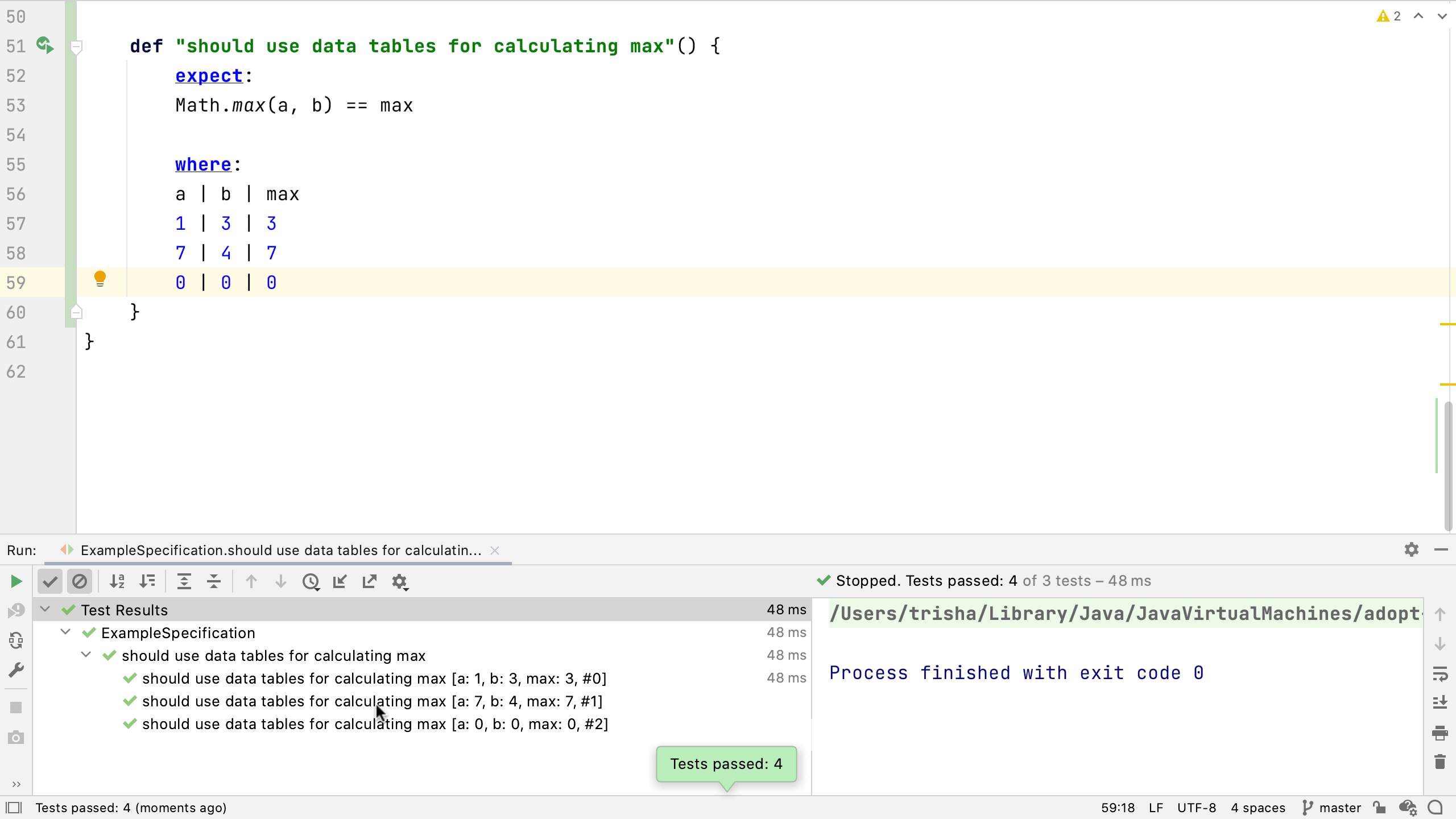There’s a passing “test” for each of the rows in the data table, described with the method name and the values for each of the input variables. If we make one of these fail we’ll see all the cases are run and one of them fails.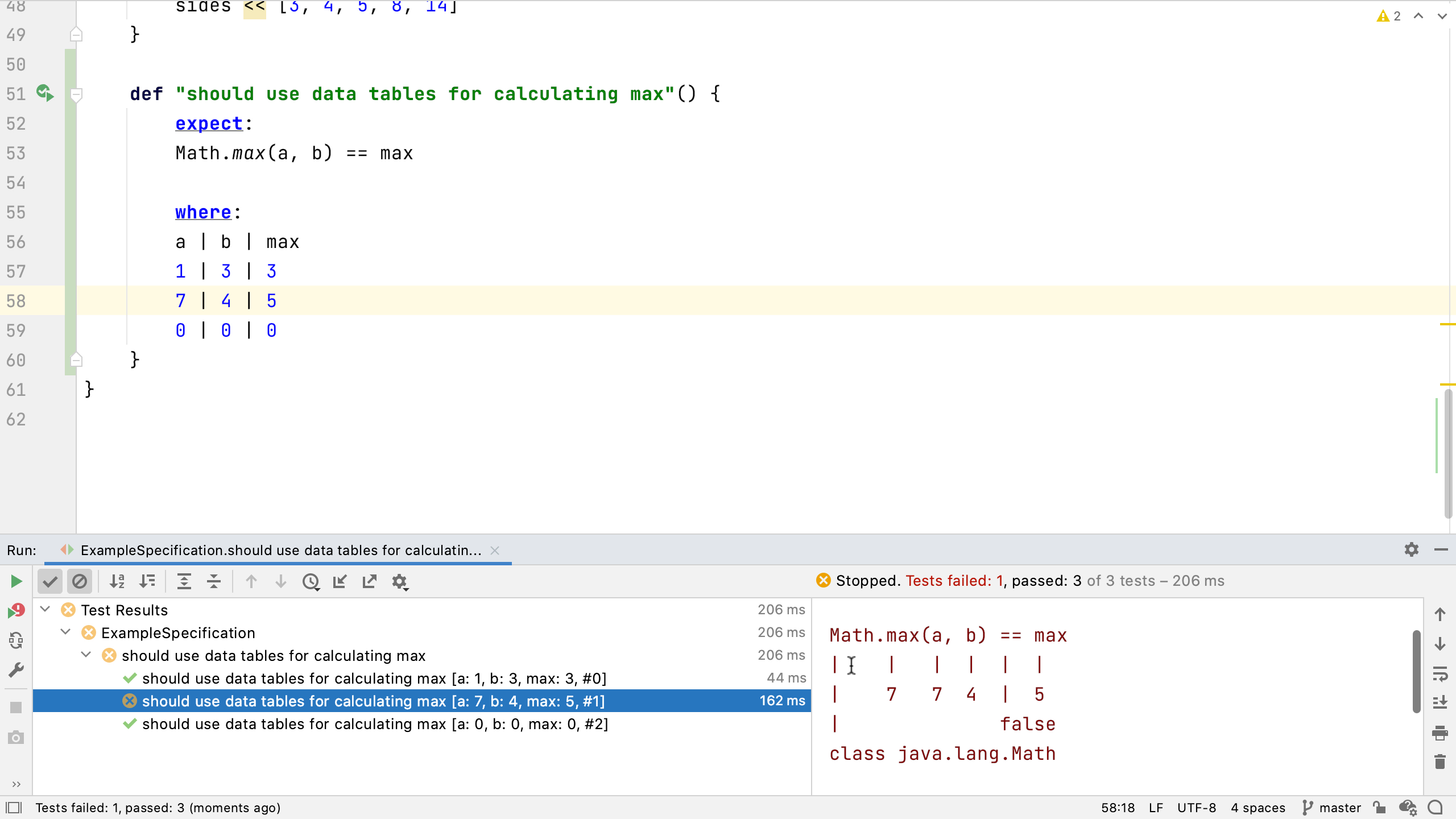Spock’s power assertions show the results of calculations, all the input values, and the comparison that failed. We can use this to fix the problem.

```Condition not satisfied:

Math.max(a, b) == max
|    |   |  |  |  |
|    7   7  4  |  5
|              false
class java.lang.Math```

We can make these data tables more readable by creating a separator between input and output columns. Try using IntelliJ IDEA’s clone caret feature – put the caret next to the `|` between `b` and `max` and press (macOS) or Ctrl (Windows/Linux) twice, keeping it held down on the second press, and pressing the down arrow to create a second caret underneath the first – do this until you have four carets, one for each line. Now if we type a second pipe, it appears on all the lines.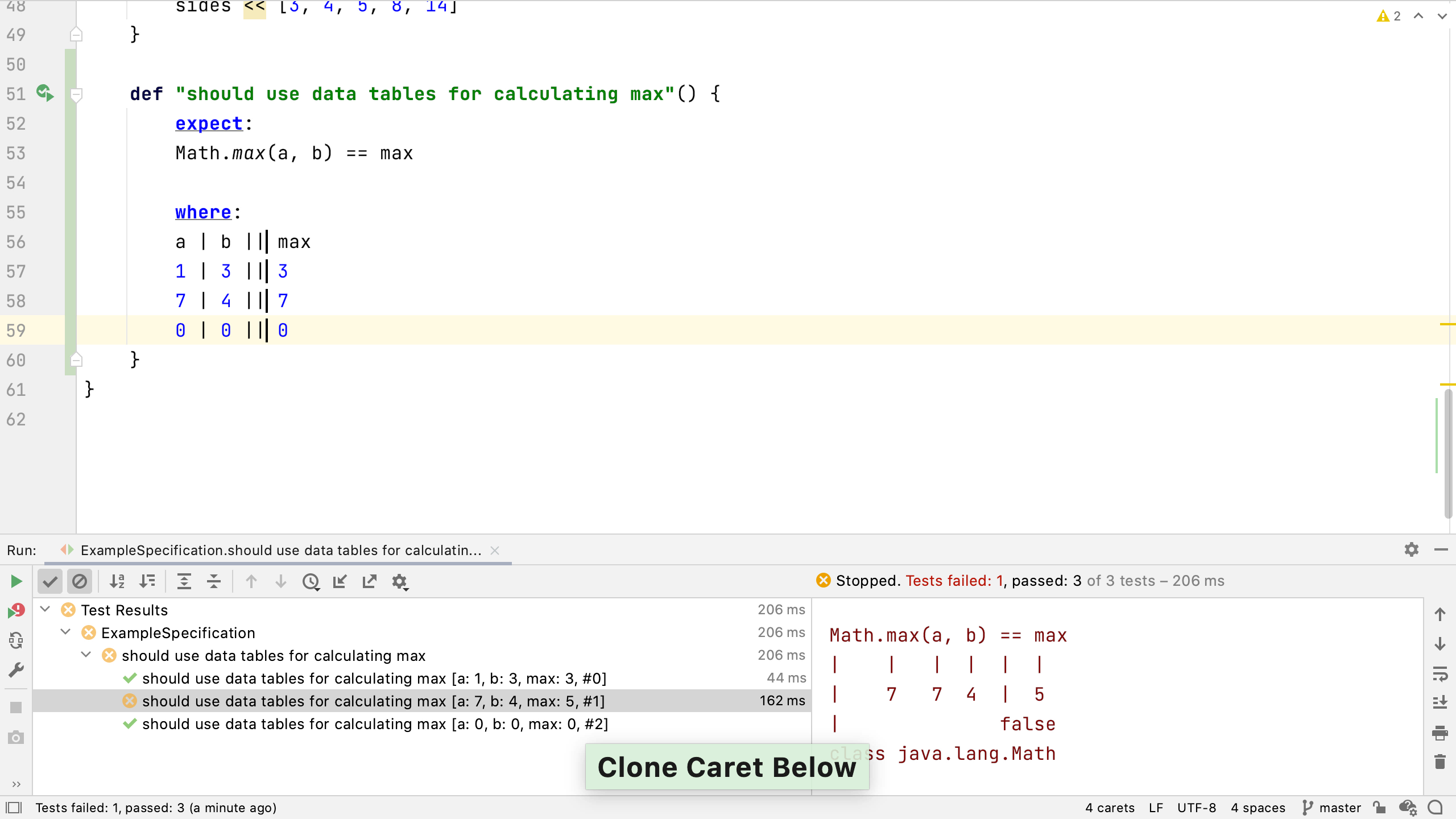This pipe doesn’t change the meaning of the test, it simply helps us to understand the table better. In our case, we’ve grouped the expected output on one side, and the inputs on the other. Run the test to make sure it works as expected.

As before, we can make the test a bit clearer by adding the names of the data variables into the test name. Now when we look at the test run, it’s really clear what’s being tested and what the expected result is.

``````def "should use data tables for calculating max. Max of #a and #b is #max"() {
expect:
Math.max(a, b) == max

where:
a | b || max
1 | 3 || 3
7 | 4 || 7
0 | 0 || 0
}``````

View steps in video

## Conclusion

In this blog, we showed how to write Spock tests that input lots of different values to a test. This means we can test and document the expected behaviour of the code under many conditions. Now you know how to:

• Use data pipes to create a set of values to use as inputs to a test
• Design data tables to create more complex sets of input and output data

Spock has much more to offer than this, stay tuned for further blog posts, watch the full video, or take a look at the excellent reference documentation.